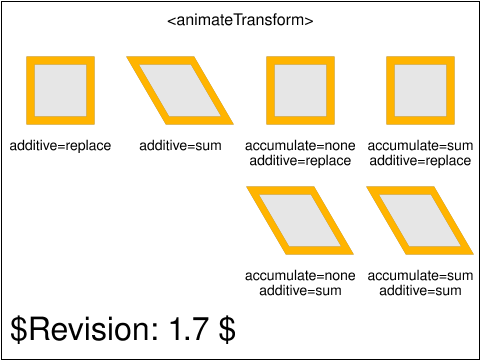Tests that contain the draft-watermark are under development and may be incorrectly testing a feature.

# animate-elem-81-t.svg## Operator Script

Run the test. No interaction required.

## Pass Criteria

The test is passed if:

• the scale of the upper leftmost grey rectangle is smoothly animated over the course of 5 seconds to completely fill the upper leftmost yellow rectangle
• the scale and rotation of the second upper grey rectangle from the left is smoothly animated over the course of 5 seconds to fill the second upper yellow rectangle from the left
• the scale of the upper third grey rectangle from the left is smoothly animated over the course of 2.5 seconds to completely fill the upper third yellow rectangle from the left, and then repeated once so that at time t=5 seconds it completely fills the same yellow rectangle
• the scale of the upper rightmost grey rectangle is smoothly animated over the course of 5 seconds to completely fill the upper rightmost yellow rectangle
• the scale of the lower leftmost grey rectangle is smoothly animated over the course of 2.5 seconds to completely fill the lower leftmost yellow rectangle, and then repeated once so that at time t=5 seconds it completely fills the same yellow rectangle
• the scale of the lower rightmost grey rectangle is smoothly animated over the course of 5 seconds to completely fill the lower rightmost yellow rectangle

## Test Description

This test tests the operation of the animateTransform's additive behavior.

The first two rectangles, on the top row, show the effect of the additive attribute on animateTransform. The left-most animateTransforms have their additive attribute set to replace, so the effect of the two transform animation is as if only the highest priority one applied because it replaces the underlying value. The second animateTransforms (from left to right) have their additive attribute set to sum, which means the transforms they produce are concatenated.

The last two rectangles, on the top row, show the effect of the accumulate attribute on animateTransform. For the left one (third on the row, from left to right), the accumulate attribute is set to none. There are two repeats for the animateTransform. Therefore, the transform goes twice from a scale(1,1) to a scale(2,2). For the right-most animateTransform, the accumulate attribute is set to sum. There are two repeats and the animation goes from scale(0,0) to scale(2,2) for the first iteration and then from scale(2,2) to scale(4,4) (because the result of the second iteration is added to the scale(2,2) result of the previous, first iteration).

The rectangles on the bottom row show the combination of additive and cumulative behavior. The left rectangle's animateTransform accumulate behavior is set to none but its additive behavior is set to sum. Therefore, the transform's underlying value (skewX(30)) is always pre-concatenated to the animateTransform's result, which goes from "skewX(30) scale(1,1)" to "skewX(30) scale(2,2)" in each of its two iterations. The right rectangle's animateTransform accumulate behavior is set to sum and the additive behavior is also set to sum. Therefore, the transform's underlying value is always pre-concatenated, and repetitions of the scale animation get added together. Consequently, the transform goes from "skewX(30) scale(0,0)" to "skewX(30) scale(2,2)" for the first iteration and then from "skewX(30) scale(2,2)" to "skewX(30) scale(4,4)" for the second iteration.